YruRU Method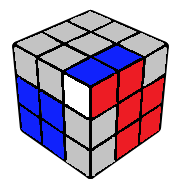(U2) R U2 R'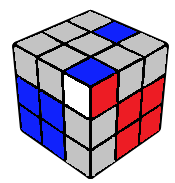(U') R U R' U' R U2 R'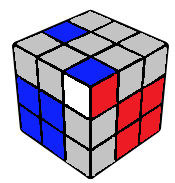(U') R U2 R' U' R U2 R'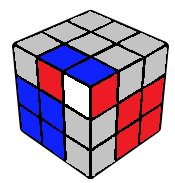R U2 R' U R U R' U R U' R'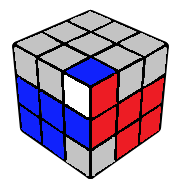(U') R U' R' U' R U2 R'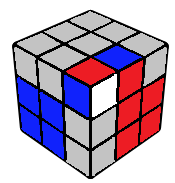(U') R U' R' U R U R'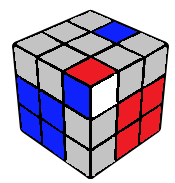R U R'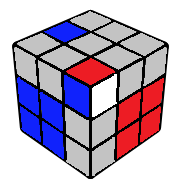(U2) R U' R' U' R U R'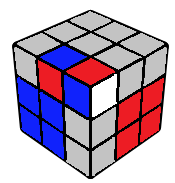R U' R' U R U' R' U2 R U' R'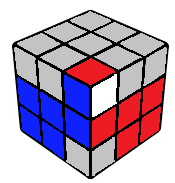(U) R U R' U2 R U R'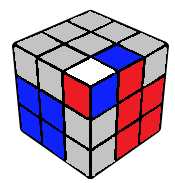R U2 R' U' R U R'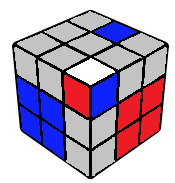(U) R U2 R' U R U' R'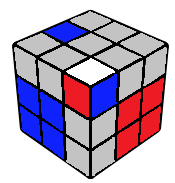(U2) R U R' U R U' R'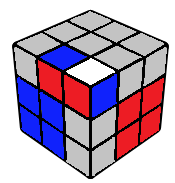R U' R' U' R U R' U2 R U R'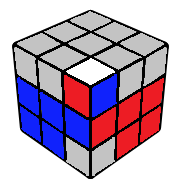R U R' U' R U R' U' R U R'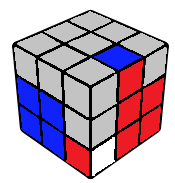R U R' U' R U R'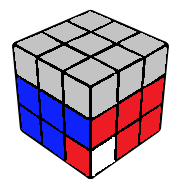R U2 R' U R U' R' U R U R'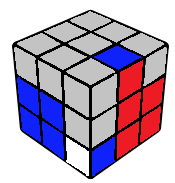R U' R' U R U' R'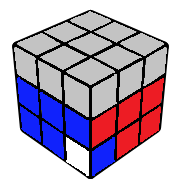R U R' U' R U2 R' U' R U R'(U2) R' U' R' U' R U R U R

Average movecount: 7.5

Average double turns: 0.9

Algorithms: 20

This is an exhaustive list of all possible last corner-edge pair cases (upto AUF) possible after square. The general theme is to bring both pieces in the top layer, separate them, set them up for a 3 move insert, and inserting them.

Note that the algorithms can be mirrored from the back in case the square is made in front, and similar transformations can be made even when using these in conjunction with advanced techniques. In case you have chosen to offset the square before beginning the pair, aim for a built pair, which will save you a move overall.